## Main

Symmetries and respective conservation laws play a central role in developing our understanding of strongly interacting states of matter. This is the case, in particular, for many systems of current interest, ranging from quantum-critical states in solids and ultracold atomic gases to quark–gluon plasmas1,2,3,4, which share common long-wavelength behaviour originating from the fundamental symmetries of spacetime. The ensuing energy and momentum conservation laws take the centre stage in these developments, defining hydrodynamics that reveals the universal collective behaviour. Powerful approaches based on conformal field theory and anti-de Sitter/conformal field theory (AdS/CFT) duality grant the well-established notions of fluid mechanics, such as viscosity and vorticity, an entirely new dimension13,14.

Despite their prominence and new paradigmatic role, viscous flows in strongly correlated systems have so far lacked directly verifiable macroscopic transport signatures. Surprisingly, this has been the case even for condensed matter systems where a wide variety of experimental techniques is available to probe collective behaviours. Identifying a signature that would do to viscous flows what zero electrical resistance did to superconductivity has remained an outstanding problem. The goal of this article is to point out that vorticity generated in viscous flows leads to a unique macroscopic transport behaviour that can serve as an unambiguous diagnostic of the viscous regime. Namely, we predict that vorticity of the shear flows generated by viscosity can result in a backflow of electrical current that can run against the applied field (see Fig. 1). The resulting negative nonlocal voltage therefore provides a clear signature of the collective viscous behaviour. Associated with it are characteristic sign-changing spatial patterns of electric potential (see Figs 1 and 2) which can be used to directly image vorticity and shear flows with modern scanning capacitance microscopy techniques15.Figure 1: Current streamlines and potential map for viscous and ohmic flows.

The negative electrical response, which is illustrated in Fig. 1, originates from the basic properties of shear flows. We recall that the collective behaviour of viscous systems results from momenta rapidly exchanged in carrier collisions while maintaining the net momentum conserved. Because momentum remains a conserved quantity collectively, it gives rise to a hydrodynamic momentum transport mode. Namely, momentum flows in space, diffusing transversely to the source–drain current flow and away from the nominal current path. A shear flow established as a result of this process generates vorticity and (for an incompressible fluid) a backflow in the direction reverse to the applied field. Such a complex and manifestly non-potential flow pattern has a direct impact on the electrical response, producing a reverse electric field acting opposite to the field driving the source–drain current (see Fig. 2). This results in a negative nonlocal resistance which persists even in the presence of fairly significant ohmic currents (see Fig. 2).

Attempts to connect electron theory with fluid mechanics have a long and interesting history, partially summarized in refs 8,16,17. Early work on the viscosity of Fermi liquids made a connection with ultrasound damping18. Subsequently, Gurzhi introduced an electronic analogue of Poiseuille flow19. Related temperature-dependent phenomena in nonlinear transport were observed by deJong and Molenkamp16. Recent developments started with the theory of a hydrodynamic, collision-dominated quantum-critical regime advanced by Damle and Sachdev1. Andreev et al. argued that hydrodynamic contributions can dominate resistivity in systems with a large disorder correlation length7. Forcella et al. predicted that electron viscosity can impact electromagnetic field penetration in a striking way8. Davison et al. linked electron viscosity to linear resistivity of the normal state of the copper oxides20.

As a parallel development, recently there has been a surge of interest in the electron viscosity of graphene5,6,9,21,22. Quantum-critical behaviour is predicted to be particularly prominent in graphene10,11,12. Electron interactions in graphene are strengthened near charge neutrality owing to the lack of screening at low carrier densities12,23. As a result, carrier collisions are expected to dominate transport in pristine graphene for a wide range of temperatures and dopings24. Furthermore, estimates of electronic viscosity near charge neutrality yield one of the lowest known viscosity-to-entropy ratios, which approaches the universal AdS/CFT bound5.

Despite the general agreement that graphene holds the key to electron viscosity, experimental progress has been hampered by the lack of easily discernible signatures in macroscopic transport. Several striking effects have been predicted, such as vortex shedding in the pre-turbulent regime induced by a strong current6, as well as nonstationary flow in a ‘viscometer’ comprised of an a.c.-driven Corbino disc9. These proposals, however, rely on fairly complex a.c. phenomena originating from high-frequency dynamics in the electron system. In each of these cases, as well as in those of refs 8,20, a model-dependent analysis was required to delineate the effects of viscosity from ‘extraneous’ contributions. In contrast, the nonlocal d.c. response considered here is a direct manifestation of the collective momentum transport mode which underpins viscous flow, therefore providing an unambiguous, almost textbook, diagnostic of the viscous regime.

A nonlocal electrical response mediated by chargeless modes was found recently to be uniquely sensitive to quantities which are not directly accessible in electrical transport measurements, in particular spin currents and valley currents25,26,27. In a similar manner, the nonlocal response discussed here gives a diagnostic of viscous transport, which is more direct and powerful than any approaches based on local transport.

There are several aspects of the electron system in graphene that are particularly well suited for studying electronic viscosity. First, the momentum-nonconserving Umklapp processes are forbidden in two-body collisions because of graphene’s crystal structure and symmetry. This ensures the prominence of momentum conservation and associated collective transport. Second, although carrier scattering is weak away from charge neutrality, it can be enhanced by several orders of magnitude by tuning the carrier density to the neutrality point. This allows one to cover the regimes of high and low viscosity, respectively, in a single sample. Last, the two-dimensional structure and atomic thickness makes the electronic states in graphene fully exposed and amenable to sensitive electric probes.

To show that the timescales are favourable for the hydrodynamical regime, we will use parameter values estimated for pristine graphene samples which are almost defect free, such as freestanding graphene28. Kinematic viscosity can be estimated as the momentum diffusion coefficient ν ≈ (1/2)vF2γee−1, where γee is the carrier–carrier scattering rate, and vF = 106 m s−1 for graphene. According to Fermi-liquid theory, this rate behaves as γee (kBT)2/EF in the degenerate limit (that is, away from charge neutrality), which leads to large ν values. Near charge neutrality, however, the rate γee grows and ν approaches the AdS/CFT limit—namely s/4πkB, where s is entropy density. Refs 12,23 estimate this rate as γee2kBT/, where α is the interaction strength. For T = 100 K, assuming EF = 0 and approximating the prefactor as A ≈ 1 (refs 12,23), this predicts characteristic times as short as γee−1 ≈ 80 fs. Disorder scattering can be estimated from the measured mean free path values, which reach a few microns at large doping29. Using the momentum relaxation rate square-root dependence on doping, γp n−1/2, and estimating it near charge neutrality, n 1010 cm−2, gives times γp−1 0.5 ps which are longer than the values γee−1 estimated above. The inequality γp γee justifies our hydrodynamical description of transport.

Momentum transport in the hydrodynamic regime is described by the continuity equation for momentum density,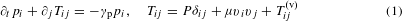where Tij is the momentum flux tensor, P and μ are pressure and mass density, and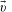is the carrier drift velocity. The quantity γp, introduced above, describes electron-lattice momentum relaxation due to disorder or phonons, which we will assume to be small compared to the carrier scattering rate. We can relate pressure to the electrochemical potential ϕ through P = $e{\int }_{{n}_{0}}^{n}\Phi \left(n\text{'}\right)\text{d}n\text{'}$. Here we work at degeneracy, EF kBT, ignoring the entropic/thermal contributions, and approximating Pe(nn0)ϕ, with n the particle number density. While carrier scattering is suppressed at degeneracy as compared to its value at EF = 0, here we assume that the carrier-carrier scattering remains faster than the disorder scattering, as required for the validity of hydrodynamics. Viscosity contributes to the momentum flux tensor through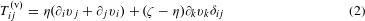where η and ζ are the first and second viscosity coefficients. For drift velocities smaller than plasmonic velocities, transport in charged systems is described by an incompressible flow with a divergenceless velocity field, ivi = 0. In this work, we consider the limit of low Reynolds number, μvivj η(ivj + jvi), such that the role of viscosity is most prominent. At linear order in, we obtain an electronic Navier–Stokes equationThis equation describes momentum transport: imparted by the external field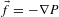, momentum flows to the system boundary where dissipation takes place. It is therefore important to endow equation (3) with suitable boundary conditions. In fluid mechanics this is described by the no-slip boundary condition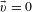. We use a slightly more general boundary condition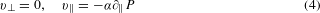where the subscripts and indicate the velocity and derivative components normal and tangential to the boundary. The second relation in equation (4) generalizes the no-slip condition to account for non-hydrodynamical effects in the boundary layer on the scales l = v/γee. The model (4), equipped with the parameter α, provides a convenient way to assess the robustness of our predictions.

It is instructive to consider a current flowing down a long strip of a finite width. A steady viscous flow features a non-uniform profile in the strip cross-section governed by the momentum flow to the boundary. Equation (3), applied to a strip 0 < y < w, yields (− η∂y2 + γpmn)v(y) = enE, where v(y) and E are the drift velocity and electric field directed along the strip (and m is an effective mass defined through the relation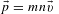). Setting α and γp to zero for simplicity, we find a parabolic profile v(y) = Ay(wy), where A = neE/2η and η = mnν. The nonzero shear yv = A(w − 2y) describes momentum flow to the boundary. The net current I = ∫ 0wnev(y′)dy′ = (n2e2/12η)w3E, scaling as a cube of the strip width, is the electronic analogue of the Poiseuille law. Being distinct from the linear scaling I wE in the ohmic regime, the cubic scaling can in principle be used to identify the viscous regime. It is interesting to put the current–field relation in a ‘Drude’ form using kinematic viscosity: I = (ne2τw/m)wE, with τw = w2/12ν being an effective scattering time. Evaluating the latter as τw ≈ (1/6)(w/vF)2γee, we find values that, for realistic system parameters, can greatly exceed the naive estimate γp−1 = w/vF based on the ballistic transport picture. This remarkable observation was first made by Gurzhi, see ref. 19.

Next, we proceed to analyse the nonlocal response in a strip with transverse current injected and drained through a pair of contacts, as pictured in Fig. 1. Unlike the above case of longitudinal current, here the potential profile is not set externally but must be obtained from equation (3). The analysis is facilitated by introducing a stream function via, which solves the incompressibility condition. At first we will completely ignore the ohmic effects, setting α and γp to zero as above, which leads to a biharmonic equation (x2 + y2)2ψ = 0 with the boundary conditions vx = 0, nevy = (x) for y = 0, w. Using a Fourier transform in x, we write ψ(x, y) = (2π)−1 ∫ dkeikxψk(y) and then determine ψk(y) separately for each k (see Supplementary Information). Inverting the Fourier transform gives the stream function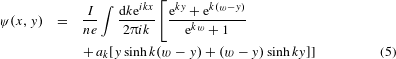where we defined ak = ktanh(kw/2)/(kw + sinhkw). Contours (isolines) of ψ give the streamlines for the flow shown in Fig. 1. Although most of them are open lines connecting source and drain, some streamlines form loops. The latter define vortices occurring on both sides of the current path. Numerically, we find that vortex centres are positioned very close to x = ± w (see Supplementary Information).

We can now explore the electrical potential of the viscous flow. The latter can be found directly from ψ(x, y), giving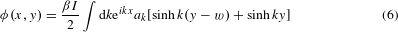where we defined β = 2η/(πn2e2) (see Supplementary Information). As illustrated in Fig. 1, equation (6) predicts a peculiar sign-changing spatial dependence, with two pairs of nodal lines crossing at contacts. To understand this behaviour, we evaluate φ(x, y) explicitly in the regions near contacts (x, y) = (0,0), (0, w). Near the first contact, approximating tanh(kw/2) ≈ sgn k, sinhky ≈ (1/2)e|k|ysgn k, and so on, we find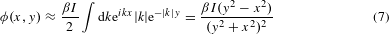(|x|, |y| w). Equation (7) predicts an inverse-square dependence versus distance from contacts and also the presence of two nodal lines running at ±45° angles relative to the nominal current path. Similar behaviour is found near the other contact, φ(x, y) ≈ − (β((wy)2x2)/((wy)2 + x2)2)I. We note that the r−2 power law dependence is much stronger than the lnr dependence expected in the ohmic regime. This, as well as multiple sign changes, provides a clear signature of a viscous flow.

The nonlocal voltage measured at a finite distance from the current leads (see schematic in Fig. 2 inset) can be evaluated as V (x) = φ(x, w) − φ(x, 0). From equation (7) we predict a voltage that is falling off as x−2 and is of a negative sign: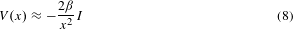(|x| w). Microscopically, negative voltage originates from a viscous shear flow which creates vorticity and backflow on both sides of the current path (see Fig. 1).

Numerically, we see that the negative response persists to arbitrarily large distances, see the ρ = 0 curve in Fig. 2. The sign change at very short x, evident in Fig. 2, arises owing to a finite contact size. We model it by replacing the delta function in the boundary condition for the current source by a Lorentzian, nevy = Ia/π(x2 + a2) at y = 0, w. After making appropriate changes in the above derivation (namely, plugging ea|k| under the integral) we find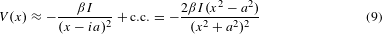This expression exhibits a sign change at x = a (representing ‘the contact edge’) and is negative for all |x| > a, that is, everywhere outside contacts (this is further discussed in Supplementary Information).

It is interesting to probe to what extent the negative response is sensitive to boundary conditions—in particular to the no-slip assumption. Extending the above analysis to the boundary conditions with nonzero α in equation (4), we find the nonlocal response of the form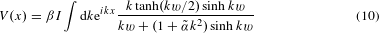where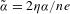. The expression under the integral represents an even function of k with a zero at k = 0 and a symmetric double-peak structure. The peaks roll off at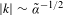, which sets an ultraviolet cutoff for the integral similar to that above for a finite-size contact model. Our numerical analysis shows that this is indeed the case, with a finite α translating into an effective contact size. In other words, the modified boundary conditions can alter the response in the proximity of the contact while rendering it unaffected at larger distances.

So far we have ignored the bulk momentum relaxation, setting γp = 0 in equation (3). We now proceed to show that the signatures of viscous flow identified above are robust in the presence of a background ohmic resistivity ρ = p/ne2 so long as it is not too strong. The dimensionless parameter which governs the respective roles of resistivity versus viscosity is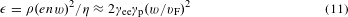For the values γee and γp quoted above, and taking w = 1 μm, one obtains ε 10. Incorporating finite resistivity in the calculation is uneventful, yielding a responsewhere q2 = k2 + εw−2, q± = q ± k (see Supplementary Information). For ε = 0 we recover the pure viscous result, which is negative, whereas for ε equation (12) gives the well-known ohmic result V (x) = (ρ/π)ln[coth(πx/2w)], which is positive. With both η and ρ nonzero, the resistance given by equation (12) is positive at large x but remains negative close to the contact. The dimensionless threshold that determines the possibility of negative electric response depends on the actual contact size. For the values used in Fig. 2 the negative response occurs even at fairly high resistivity values corresponding to ε 100.

The robustness of the negative response can be understood by noting that viscosity is the coefficient of the highest derivative term in equation (3) and thus dominates at short distances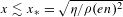. The pervasive character of the negative response, manifest in Fig. 2, will facilitate experimental detection of viscous transport. The positions of the nodes, marked by arrows in Fig. 2, vary with the ratio ρ/η, which provides a convenient way to directly measure the electronic viscosity.

The hydrodynamic transport regime also features interesting thermal effects. At leading order in the flow velocity those are dominated by convective heat transfer, described by a proportionality relation between entropy flux and flow velocity v (to be discussed elsewhere). At higher order in v, besides the conventional Joule heating, vorticity of a viscous flow manifests itself in heat productionThe vorticity-induced heating pattern, shown in Fig. 3, features hot spots near contacts and cold arc-shaped elongated patches in vorticity regions. In contrast, for an ohmic flow the pattern is essentially featureless. This makes viscous flows an interesting system to explore with the nanoscale temperature scanning techniques30.